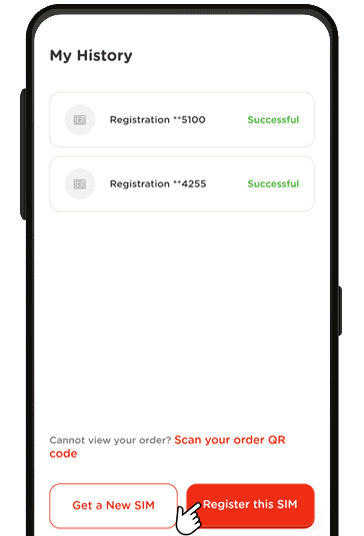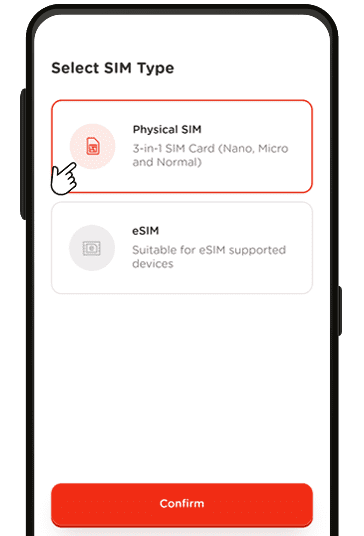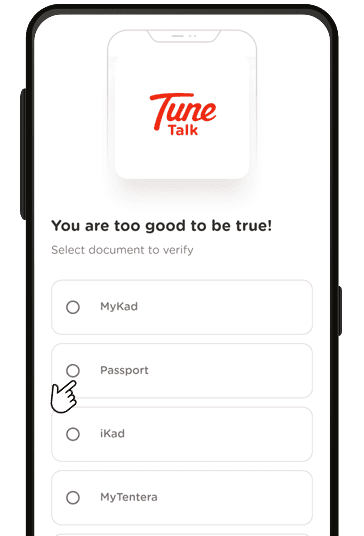## SIM IT TO ME BABY

Nothing says that you’re enjoying your holiday more than flooding the news feed with your chillin’ holiday pics!

And we’re making it easy for you by just letting you text us and TADAA!

• 1
Details
• 2
Payment
• 3
Confirmation
Name*:
(as per Passport)
Email*:
Reconfirm Email*:
Contact*:
Date of Arrival*:
Collection Options*:
For user to choose the standard shipping, the arrival date should have at least 14 days before the order date.
Postcode:*
City:*
Country:*
State:*

*I have read the Terms & Conditions and hereby agree and accept it.

All fields are required

#### Activate your Traveller SIM with the Tune Talk App

For standard shipping customers only.STEP 1
Download the Tune Talk App from your preferred App Store. Once installed, click on "Activate SIM" to get startedSTEP 2
Tap on “Register this SIMSTEP 3
Select “Get a New Number” and confirmSTEP 4
Choose "Physical SIM" and scan the SIM card barcode to activate itSTEP 5
select "Passport" and follow the app instructionsSTEP 6
Follow the app instruction to complete the face recognition
Response time: 1.6413168907166
1. select * from `rainlab_translate_attributes` where `locale` = ? and `model_id` = ? and `model_type` = ? limit 1
2. select * from `rainlab_translate_attributes` where `locale` = ? and `model_id` = ? and `model_type` = ? limit 1
3. select * from `rainlab_translate_attributes` where `locale` = ? and `model_id` = ? and `model_type` = ? limit 1
4. select * from `rainlab_translate_attributes` where `locale` = ? and `model_id` = ? and `model_type` = ? limit 1
5. select * from `rainlab_translate_attributes` where `locale` = ? and `model_id` = ? and `model_type` = ? limit 1
6. select * from `rainlab_translate_attributes` where `locale` = ? and `model_id` = ? and `model_type` = ? limit 1
7. select * from `rainlab_translate_attributes` where `locale` = ? and `model_id` = ? and `model_type` = ? limit 1
8. select * from `rainlab_translate_attributes` where `locale` = ? and `model_id` = ? and `model_type` = ? limit 1
9. select * from `rainlab_translate_attributes` where `locale` = ? and `model_id` = ? and `model_type` = ? limit 1
10. select * from `rainlab_translate_attributes` where `locale` = ? and `model_id` = ? and `model_type` = ? limit 1
11. select * from `rainlab_translate_attributes` where `locale` = ? and `model_id` = ? and `model_type` = ? limit 1
12. select * from `rainlab_translate_attributes` where `locale` = ? and `model_id` = ? and `model_type` = ? limit 1
13. select * from `rainlab_translate_attributes` where `locale` = ? and `model_id` = ? and `model_type` = ? limit 1
14. select * from `rainlab_translate_attributes` where `locale` = ? and `model_id` = ? and `model_type` = ? limit 1
15. select * from `rainlab_translate_attributes` where `locale` = ? and `model_id` = ? and `model_type` = ? limit 1
16. select * from `rainlab_translate_attributes` where `locale` = ? and `model_id` = ? and `model_type` = ? limit 1
17. select * from `rainlab_translate_attributes` where `locale` = ? and `model_id` = ? and `model_type` = ? limit 1
18. select * from `rainlab_translate_attributes` where `locale` = ? and `model_id` = ? and `model_type` = ? limit 1
19. select * from `rainlab_translate_attributes` where `locale` = ? and `model_id` = ? and `model_type` = ? limit 1
20. select * from `rainlab_translate_attributes` where `locale` = ? and `model_id` = ? and `model_type` = ? limit 1
21. select * from `rainlab_translate_attributes` where `locale` = ? and `model_id` = ? and `model_type` = ? limit 1
22. select * from `rainlab_translate_attributes` where `locale` = ? and `model_id` = ? and `model_type` = ? limit 1
23. select * from `rainlab_translate_attributes` where `locale` = ? and `model_id` = ? and `model_type` = ? limit 1
24. select * from `rainlab_translate_attributes` where `locale` = ? and `model_id` = ? and `model_type` = ? limit 1
25. select * from `rainlab_translate_attributes` where `locale` = ? and `model_id` = ? and `model_type` = ? limit 1
26. select * from `rainlab_translate_attributes` where `locale` = ? and `model_id` = ? and `model_type` = ? limit 1
27. select * from `rainlab_translate_attributes` where `locale` = ? and `model_id` = ? and `model_type` = ? limit 1
28. select * from `rainlab_translate_attributes` where `locale` = ? and `model_id` = ? and `model_type` = ? limit 1
29. select * from `rainlab_translate_attributes` where `locale` = ? and `model_id` = ? and `model_type` = ? limit 1
30. select * from `rainlab_translate_attributes` where `locale` = ? and `model_id` = ? and `model_type` = ? limit 1
31. select * from `rainlab_translate_attributes` where `locale` = ? and `model_id` = ? and `model_type` = ? limit 1
32. select * from `rainlab_translate_attributes` where `locale` = ? and `model_id` = ? and `model_type` = ? limit 1
33. select * from `rainlab_translate_attributes` where `locale` = ? and `model_id` = ? and `model_type` = ? limit 1
34. select * from `rainlab_translate_attributes` where `locale` = ? and `model_id` = ? and `model_type` = ? limit 1
35. select * from `rainlab_translate_attributes` where `locale` = ? and `model_id` = ? and `model_type` = ? limit 1
36. select * from `rainlab_translate_attributes` where `locale` = ? and `model_id` = ? and `model_type` = ? limit 1
37. select * from `rainlab_translate_attributes` where `locale` = ? and `model_id` = ? and `model_type` = ? limit 1
38. select * from `rainlab_translate_attributes` where `locale` = ? and `model_id` = ? and `model_type` = ? limit 1
39. select * from `rainlab_translate_attributes` where `locale` = ? and `model_id` = ? and `model_type` = ? limit 1
40. select * from `rainlab_translate_attributes` where `locale` = ? and `model_id` = ? and `model_type` = ? limit 1
41. select * from `rainlab_translate_attributes` where `locale` = ? and `model_id` = ? and `model_type` = ? limit 1
42. select * from `rainlab_translate_attributes` where `locale` = ? and `model_id` = ? and `model_type` = ? limit 1
43. select * from `rainlab_translate_attributes` where `locale` = ? and `model_id` = ? and `model_type` = ? limit 1
44. select * from `rainlab_translate_attributes` where `locale` = ? and `model_id` = ? and `model_type` = ? limit 1
45. select * from `rainlab_translate_attributes` where `locale` = ? and `model_id` = ? and `model_type` = ? limit 1
46. select * from `rainlab_translate_attributes` where `locale` = ? and `model_id` = ? and `model_type` = ? limit 1
47. select * from `rainlab_translate_attributes` where `locale` = ? and `model_id` = ? and `model_type` = ? limit 1
48. select * from `rainlab_translate_attributes` where `locale` = ? and `model_id` = ? and `model_type` = ? limit 1
49. select * from `rainlab_translate_attributes` where `locale` = ? and `model_id` = ? and `model_type` = ? limit 1
50. select * from `rainlab_translate_attributes` where `locale` = ? and `model_id` = ? and `model_type` = ? limit 1
51. select * from `rainlab_translate_attributes` where `locale` = ? and `model_id` = ? and `model_type` = ? limit 1
52. select * from `rainlab_translate_attributes` where `locale` = ? and `model_id` = ? and `model_type` = ? limit 1
53. select * from `rainlab_translate_attributes` where `locale` = ? and `model_id` = ? and `model_type` = ? limit 1
54. select * from `rainlab_translate_attributes` where `locale` = ? and `model_id` = ? and `model_type` = ? limit 1
55. select * from `rainlab_translate_attributes` where `locale` = ? and `model_id` = ? and `model_type` = ? limit 1
56. select * from `rainlab_translate_attributes` where `locale` = ? and `model_id` = ? and `model_type` = ? limit 1
57. select * from `rainlab_translate_attributes` where `locale` = ? and `model_id` = ? and `model_type` = ? limit 1
58. select * from `rainlab_translate_attributes` where `locale` = ? and `model_id` = ? and `model_type` = ? limit 1
59. select * from `rainlab_translate_attributes` where `locale` = ? and `model_id` = ? and `model_type` = ? limit 1
60. select * from `rainlab_translate_attributes` where `locale` = ? and `model_id` = ? and `model_type` = ? limit 1
61. select * from `rainlab_translate_attributes` where `locale` = ? and `model_id` = ? and `model_type` = ? limit 1
62. select * from `rainlab_translate_attributes` where `locale` = ? and `model_id` = ? and `model_type` = ? limit 1
63. select * from `rainlab_translate_attributes` where `locale` = ? and `model_id` = ? and `model_type` = ? limit 1
64. select * from `rainlab_translate_attributes` where `locale` = ? and `model_id` = ? and `model_type` = ? limit 1
65. select * from `rainlab_translate_attributes` where `locale` = ? and `model_id` = ? and `model_type` = ? limit 1
66. select * from `rainlab_translate_attributes` where `locale` = ? and `model_id` = ? and `model_type` = ? limit 1
67. select * from `rainlab_translate_attributes` where `locale` = ? and `model_id` = ? and `model_type` = ? limit 1
68. select * from `rainlab_translate_locales` where `code` = ? limit 1
69. select `tunetalk_element_h_b_groups`.*, `tunetalk_front_contentable`.`content_id` as `pivot_content_id`, `tunetalk_front_contentable`.`contentable_id` as `pivot_contentable_id` from `tunetalk_element_h_b_groups` inner join `tunetalk_front_contentable` on `tunetalk_element_h_b_groups`.`id` = `tunetalk_front_contentable`.`contentable_id` where `tunetalk_front_contentable`.`content_id` = ? and `tunetalk_front_contentable`.`contentable_type` = ?
70. select * from `tunetalk_element_hero_banners` where `tunetalk_element_hero_banners`.`group_id` = ? and `tunetalk_element_hero_banners`.`group_id` is not null order by `position` asc
71. select `tunetalk_element_t_n_groups`.*, `tunetalk_front_contentable`.`content_id` as `pivot_content_id`, `tunetalk_front_contentable`.`contentable_id` as `pivot_contentable_id` from `tunetalk_element_t_n_groups` inner join `tunetalk_front_contentable` on `tunetalk_element_t_n_groups`.`id` = `tunetalk_front_contentable`.`contentable_id` where `tunetalk_front_contentable`.`content_id` = ? and `tunetalk_front_contentable`.`contentable_type` = ?
72. select `tunetalk_support_faq_categories`.*, `tunetalk_front_contentable`.`content_id` as `pivot_content_id`, `tunetalk_front_contentable`.`contentable_id` as `pivot_contentable_id` from `tunetalk_support_faq_categories` inner join `tunetalk_front_contentable` on `tunetalk_support_faq_categories`.`id` = `tunetalk_front_contentable`.`contentable_id` where `tunetalk_front_contentable`.`content_id` = ? and `tunetalk_front_contentable`.`contentable_type` = ?
73. select * from `system_files` where `system_files`.`attachment_type` = ? and `system_files`.`attachment_id` = ? and `field` = ? and `system_files`.`attachment_id` is not null order by `sort_order` asc limit 1
74. select `id` from `tunetalk_coolplan_countries` where `iso_code_2` = ? limit 1
75. select `name` from `tunetalk_coolplan_states` where `countries_id` = ?
76. select * from `tunetalk_store_magento_store_views` where `default` = ? limit 1
77. select * from `tunetalk_coolplan_countries` order by `name` asc
78. select * from `tunetalk_store_traveller_collectoptions` order by `sort_order` asc
79. select * from `system_files` where `system_files`.`attachment_type` = ? and `system_files`.`attachment_id` = ? and `field` = ? and `system_files`.`attachment_id` is not null order by `sort_order` asc limit 1
80. select * from `system_files` where `system_files`.`attachment_type` = ? and `system_files`.`attachment_id` = ? and `field` = ? and `system_files`.`attachment_id` is not null order by `sort_order` asc limit 1
81. select * from `system_files` where `system_files`.`attachment_type` = ? and `system_files`.`attachment_id` = ? and `field` = ? and `system_files`.`attachment_id` is not null order by `sort_order` asc limit 1
82. select * from `system_files` where `system_files`.`attachment_type` = ? and `system_files`.`attachment_id` = ? and `field` = ? and `system_files`.`attachment_id` is not null order by `sort_order` asc limit 1
83. select * from `system_files` where `system_files`.`attachment_type` = ? and `system_files`.`attachment_id` = ? and `field` = ? and `system_files`.`attachment_id` is not null order by `sort_order` asc limit 1
84. select * from `system_files` where `system_files`.`attachment_type` = ? and `system_files`.`attachment_id` = ? and `field` = ? and `system_files`.`attachment_id` is not null order by `sort_order` asc limit 1
85. select * from `rainlab_translate_attributes` where `locale` = ? and `model_id` = ? and `model_type` = ? limit 1
86. select * from `rainlab_translate_attributes` where `locale` = ? and `model_id` = ? and `model_type` = ? limit 1
87. select * from `rainlab_translate_attributes` where `locale` = ? and `model_id` = ? and `model_type` = ? limit 1
88. select * from `rainlab_translate_attributes` where `locale` = ? and `model_id` = ? and `model_type` = ? limit 1
89. select * from `rainlab_translate_attributes` where `locale` = ? and `model_id` = ? and `model_type` = ? limit 1
90. select * from `rainlab_translate_attributes` where `locale` = ? and `model_id` = ? and `model_type` = ? limit 1
91. select * from `rainlab_translate_attributes` where `locale` = ? and `model_id` = ? and `model_type` = ? limit 1
92. select * from `rainlab_translate_attributes` where `locale` = ? and `model_id` = ? and `model_type` = ? limit 1
93. select * from `rainlab_translate_attributes` where `locale` = ? and `model_id` = ? and `model_type` = ? limit 1
94. select * from `rainlab_translate_attributes` where `locale` = ? and `model_id` = ? and `model_type` = ? limit 1
95. select * from `rainlab_translate_attributes` where `locale` = ? and `model_id` = ? and `model_type` = ? limit 1
96. select * from `rainlab_translate_attributes` where `locale` = ? and `model_id` = ? and `model_type` = ? limit 1
97. select * from `rainlab_translate_attributes` where `locale` = ? and `model_id` = ? and `model_type` = ? limit 1
98. select * from `rainlab_translate_attributes` where `locale` = ? and `model_id` = ? and `model_type` = ? limit 1
99. select * from `rainlab_translate_attributes` where `locale` = ? and `model_id` = ? and `model_type` = ? limit 1
100. select * from `rainlab_translate_attributes` where `locale` = ? and `model_id` = ? and `model_type` = ? limit 1
101. select * from `rainlab_translate_attributes` where `locale` = ? and `model_id` = ? and `model_type` = ? limit 1
102. select * from `rainlab_translate_attributes` where `locale` = ? and `model_id` = ? and `model_type` = ? limit 1
103. select * from `rainlab_translate_attributes` where `locale` = ? and `model_id` = ? and `model_type` = ? limit 1
104. select * from `rainlab_translate_attributes` where `locale` = ? and `model_id` = ? and `model_type` = ? limit 1
105. select * from `rainlab_translate_attributes` where `locale` = ? and `model_id` = ? and `model_type` = ? limit 1
106. select * from `rainlab_translate_attributes` where `locale` = ? and `model_id` = ? and `model_type` = ? limit 1
107. select * from `rainlab_translate_attributes` where `locale` = ? and `model_id` = ? and `model_type` = ? limit 1
108. select * from `rainlab_translate_attributes` where `locale` = ? and `model_id` = ? and `model_type` = ? limit 1
109. select * from `rainlab_translate_attributes` where `locale` = ? and `model_id` = ? and `model_type` = ? limit 1
110. select * from `rainlab_translate_attributes` where `locale` = ? and `model_id` = ? and `model_type` = ? limit 1